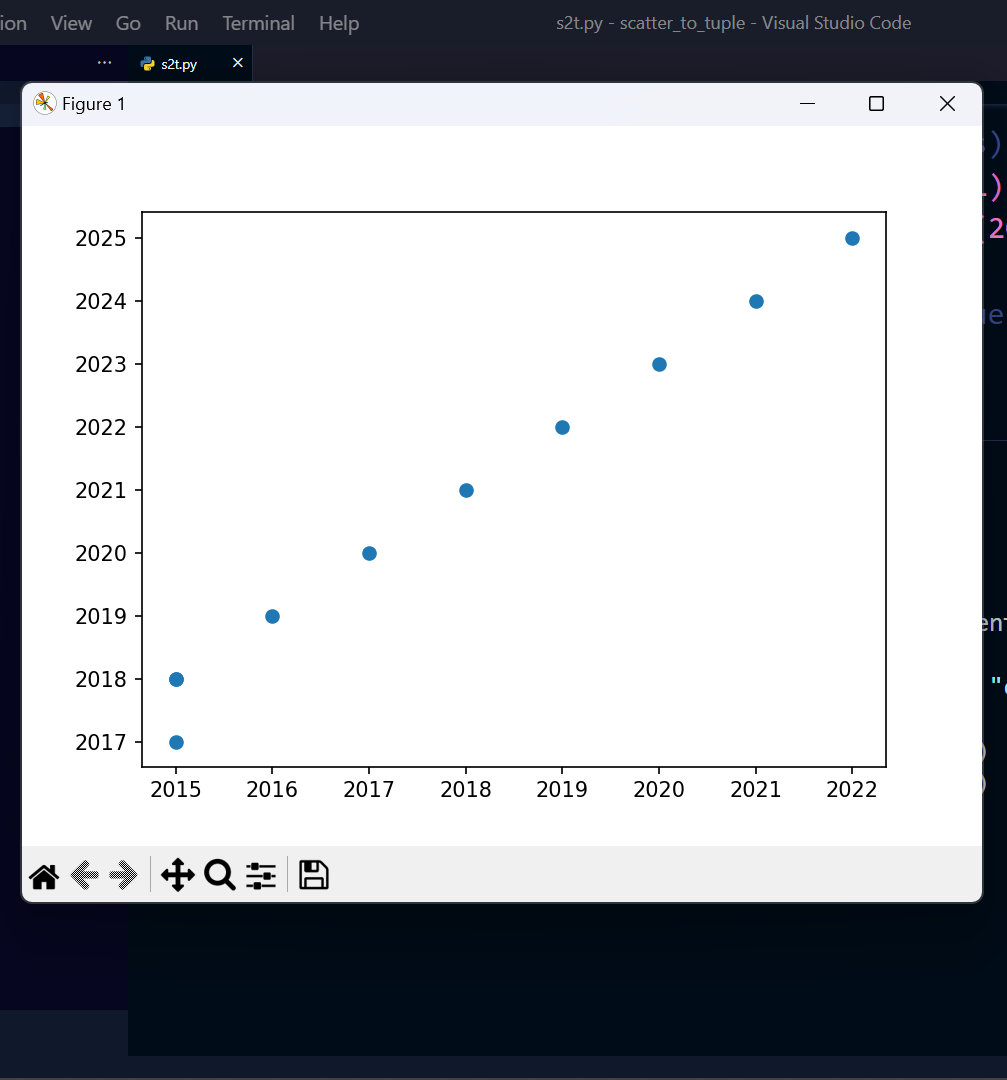Open in App
Not now

# Make Scatter Plot From Set of Points in Python Tuples

• Last Updated : 03 Jan, 2023

Now we’ll look at an example that shows how to use scatter and how scatter values can be passed to a function as a tuple argument. Assume your function takes four and five arguments each argument will be passed as a separate single data point or value to plot the scatter chart. Let’s see the implementation of it in Python.

## What is Scatter in Python?

Scatter plots are used to observe the relationship between variables and use dots to represent the relationship between them. The scatter() method in the Matplotlib library is used to draw a scatter plot. Scatter plots are widely used to represent relations among variables and how a change in one affects the other.

Syntax: matplotlib.pyplot.scatter

### Stepwise Implementation

Step 1:  Creating Tuple Data

Suppose you have two tuple values, the year in which one was admitted to engineering college and the year in which he/she will complete his/her degree. Lets store this data in the data variable.

## Python3

 `# Tuple of (Starting years and Ending years)``data ``=` `((``2015``, ``2018``), (``2015``, ``2017``), (``2018``, ``2021``),``        ``(``2020``, ``2023``), (``2017``, ``2020``), (``2016``, ``2019``),``        ``(``2015``, ``2018``), (``2019``, ``2022``), (``2022``, ``2025``),``        ``(``2021``, ``2024``))`

Step 2: Unpacking Values

Here, we’ll use the zip() function to get the start_year and end_year values. We will pass the tuple as an argument to this function, and it will extract the tuple, perform the task, and return the result.

## Python

 `# Tuple of (Starting years and Ending years)``data ``=` `((``2015``, ``2018``), (``2015``, ``2017``), (``2018``, ``2021``),``        ``(``2020``, ``2023``), (``2017``, ``2020``),``        ``(``2016``, ``2019``), (``2015``, ``2018``), (``2019``, ``2022``),``        ``(``2022``, ``2025``), (``2021``, ``2024``))` `# Using zip function and Unpacking the values``Start_year, End_year ``=` `zip``(``*``data)` `# printing the scatter values``print``(Start_year)``print``(End_year)`

Output:

```(2015, 2015, 2018, 2020, 2017, 2016, 2015, 2019, 2022, 2021)
(2018, 2017, 2021, 2023, 2020, 2019, 2018, 2022, 2025, 2024)```

Step 3: Plotting Scatter Graph

As you can see using Python Zip() to unpack the values and returns a tuple object for starting and ending years. Here * denotes that the argument is variable length. You may also use matplotlib.pyplot package and can plot scatter charts using the values in a graph using the scatter function.

## Python3

 `import` `matplotlib.pyplot as plt` `# Tuple of (Starting years and Ending years)``data ``=` `((``2015``, ``2018``), (``2015``, ``2017``),``        ``(``2018``, ``2021``), (``2020``, ``2023``), (``2017``, ``2020``),``        ``(``2016``, ``2019``), (``2015``, ``2018``), (``2019``, ``2022``),``        ``(``2022``, ``2025``), (``2021``, ``2024``))` `# Using zip function and Unpacking the values``Start_year, End_year ``=` `zip``(``*``data)` `# printing the scatter values``print``(Start_year)``print``(End_year)` `plt.scatter(Start_year, End_year)``plt.show()`

Output:My Personal Notes arrow_drop_up